# OrgChart Now Help Guide

### Audience

##### Overview

Dynamic Formulas are calculated based on the present data, and can be displayed on charts, and within chart boxes.

Dynamic formulas are helpful when doing roll-ups in Workbench. For example, if you are using a dynamic salary rollup, and you move or delete a record, the dynamic formula will automatically update.

OrgChart comes pre-loaded with Dynamic Formulas, which are distinguished from their static counterparts using an underscore:

• _Levels

• _Peers

• _Span of Control

• _Subordinates

Administrators can create custom Dynamic Formulas in the Chart Settings: Chart Builder panel.

##### Accessing the Dynamic Formula Editor

Click on the Mode Switcher icon in the Top Toolbar, and then select the Setup option. The Setup panel is displayed.

Click on the Chart Settings tile.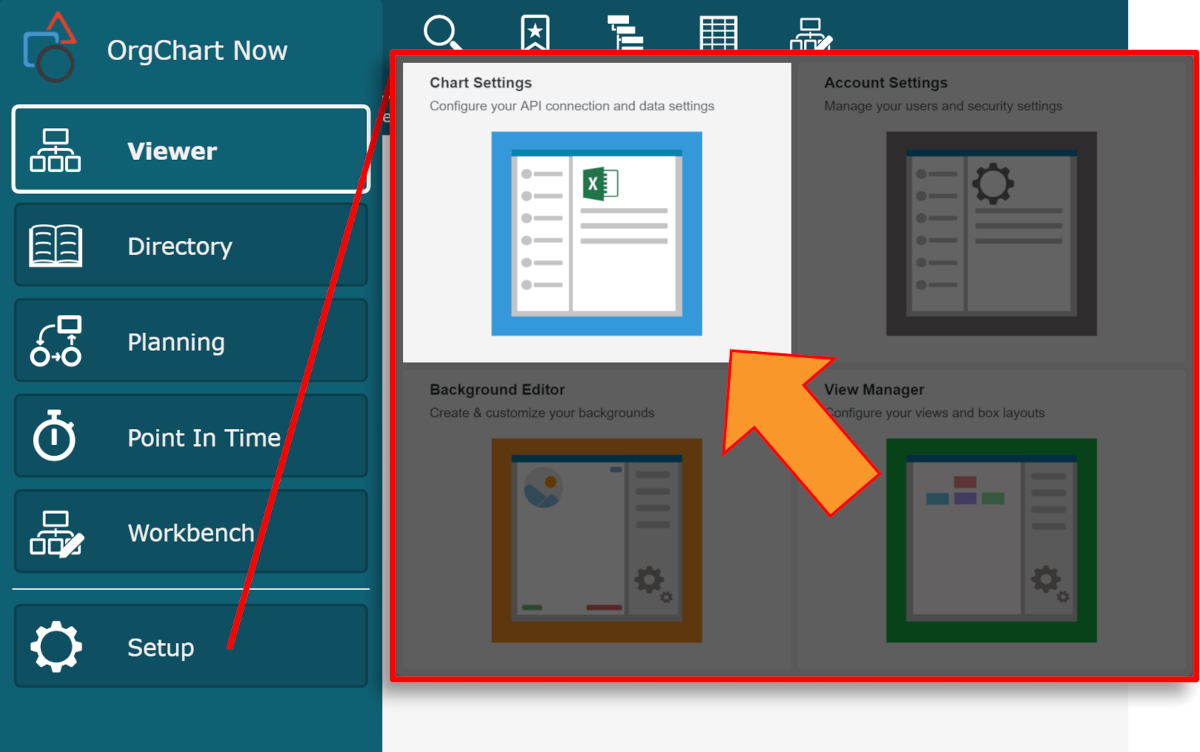Click on the Chart Builder tab from left side menu.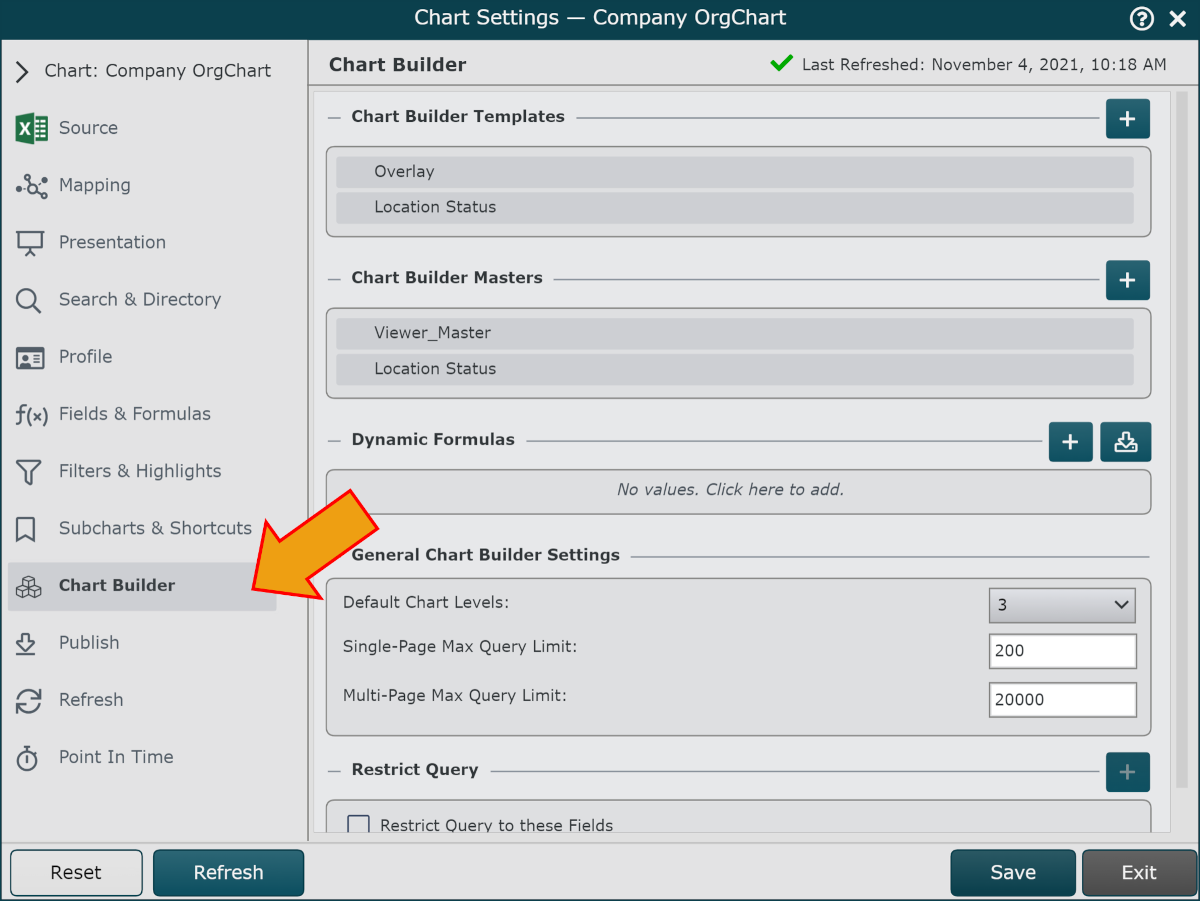Add a new Dynamic Formula using one of the following three methods:

• Click on the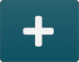icon associated with the Dynamic Formula heading.

• Click on theicon to import a Dynamic Formula from a different Master Chart.

###### Defining a New Dynamic Formula

The following example demonstrates how to create a formula that averages employee salary.

1. Click on theicon to the right of the Dynamic Formula heading.

2. Select an operator (i.e. Average) from the Formula Type dropdown menu.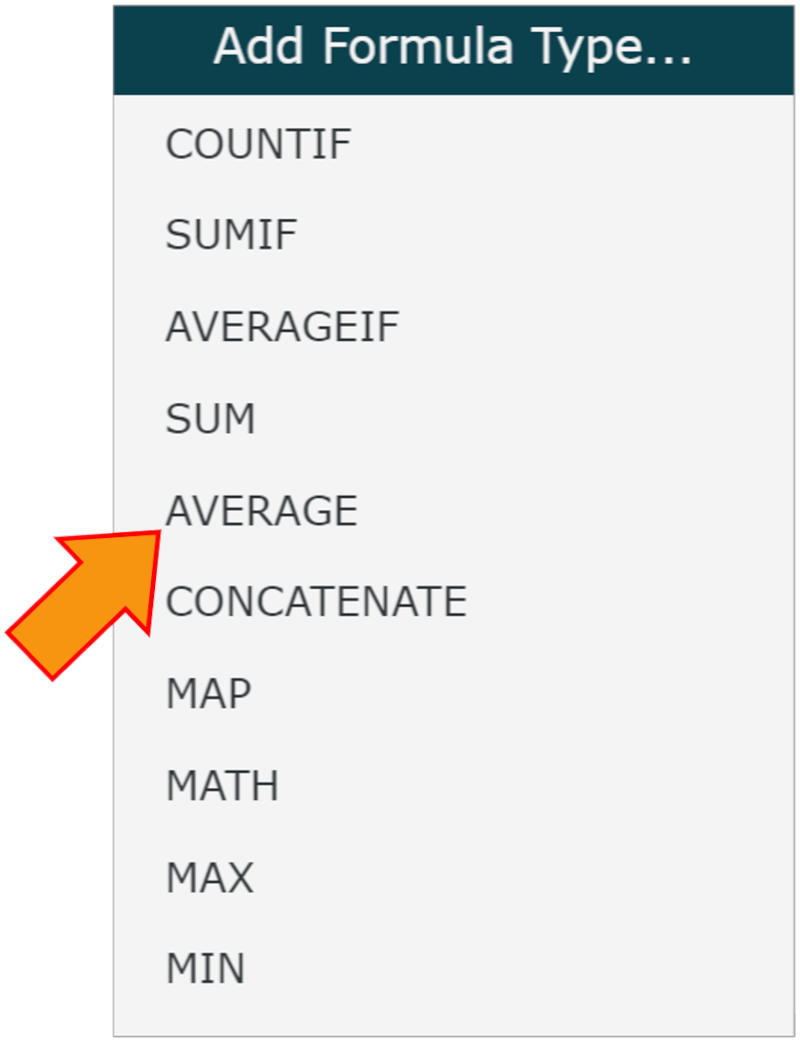3. Enter a name for the formula in the Formula Name text box (i.e. _Salary)

### Note

It is not required to use an underscore in the name of a Dynamic Formula.

4. Click on the Formula Span dropdown menu, and then select one of the following options:

• Self + Subordinates - Current record and all subordinates

• Subordinates - Subordinates of the current record

• Self + Direct Reports - Current record and the records directly beneath that record

• Direct Reports - The records directly beneath the current record

### Note

Note: When setting the conditions for this rule, you must use brackets around the field, a Comparison Operator, and then a valid string (enclosed in single or double quotations) or a number value.

5. Click on the Formula Field dropdown menu, and then select a field from your data (i.e., Salary) that you want to calculate.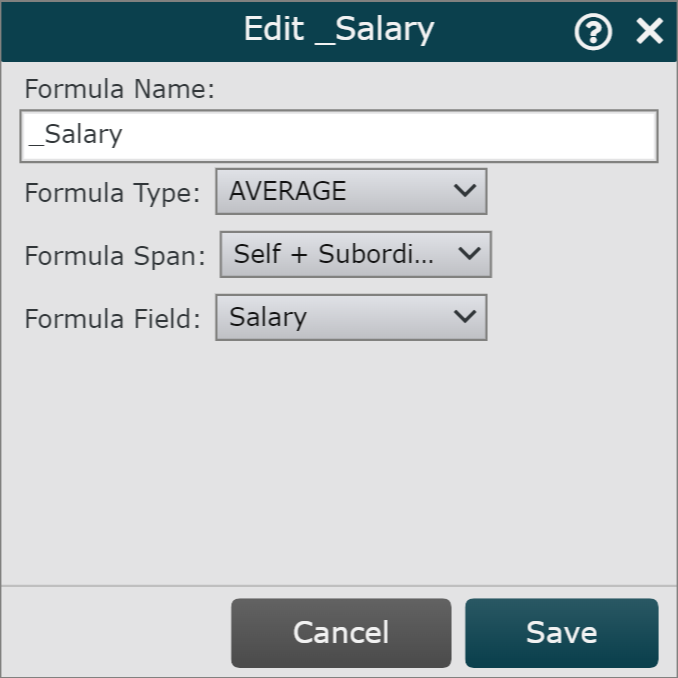6. Click Save. The formula is added to the Dynamic Formula list.

7. Click on Save, and then Refresh.

Hover over an existing Dynamic Formula to access the following additional options:CopyEditDelete
###### Available Operations

Operation

Description

CountIf

Count the values of a defined field IF they match the formula criteria

SumIf

Sum the values of a defined field IF they match the formula criteria

AverageIf

Average the values of a defined field IF they match the formula criteria

Sum

Sum the values of a defined field

Average

Average the values of a defined field

Concatenate

Concatenate the values defined by the formula rule

Map

Map a defined value when the chosen field matches the formula criteria

Math

Calculate a free-form JavaScript formula

Max

Max value in an org chart branch for a specified field

Min

Min value in an org chart branch for a specified field

###### Comparison & Logic Operators

Operator

Description

==

Equal to

!=

Not equal to

>

Greater than

>=

Great than or equal to

<

Less than

<=

Less than or equal to

?

Ternary operator

&&

Logical and

||

Logical or

!

Logical not